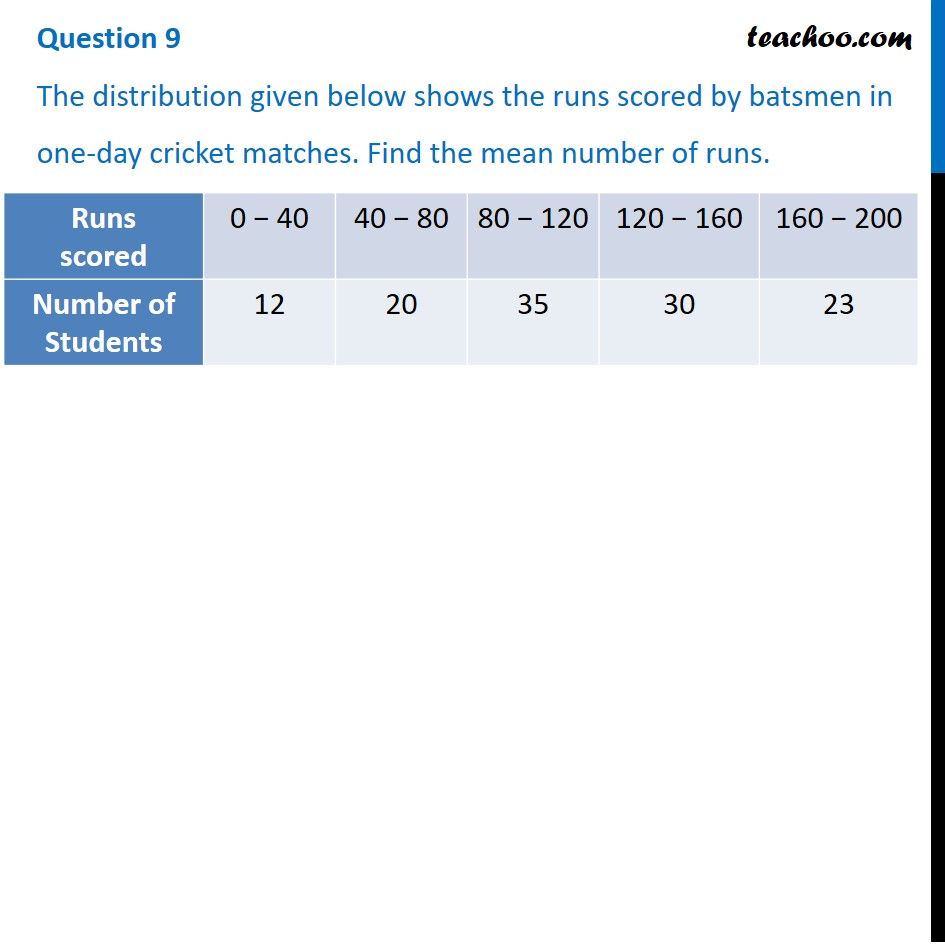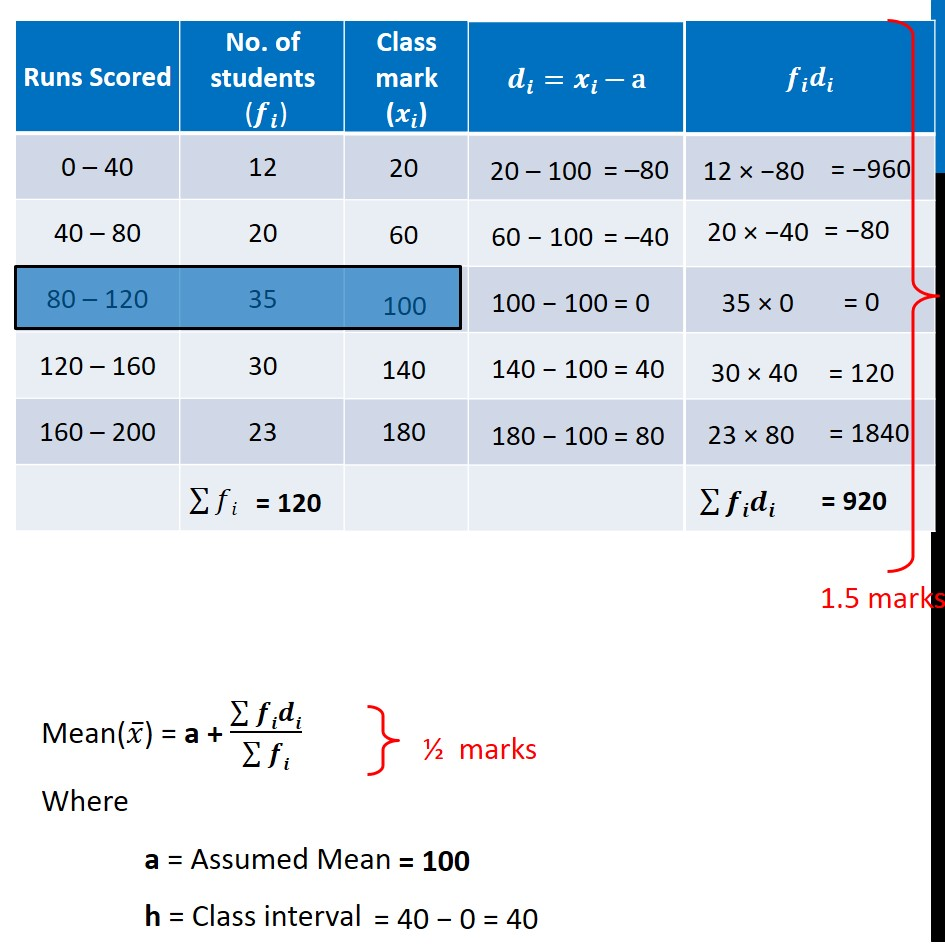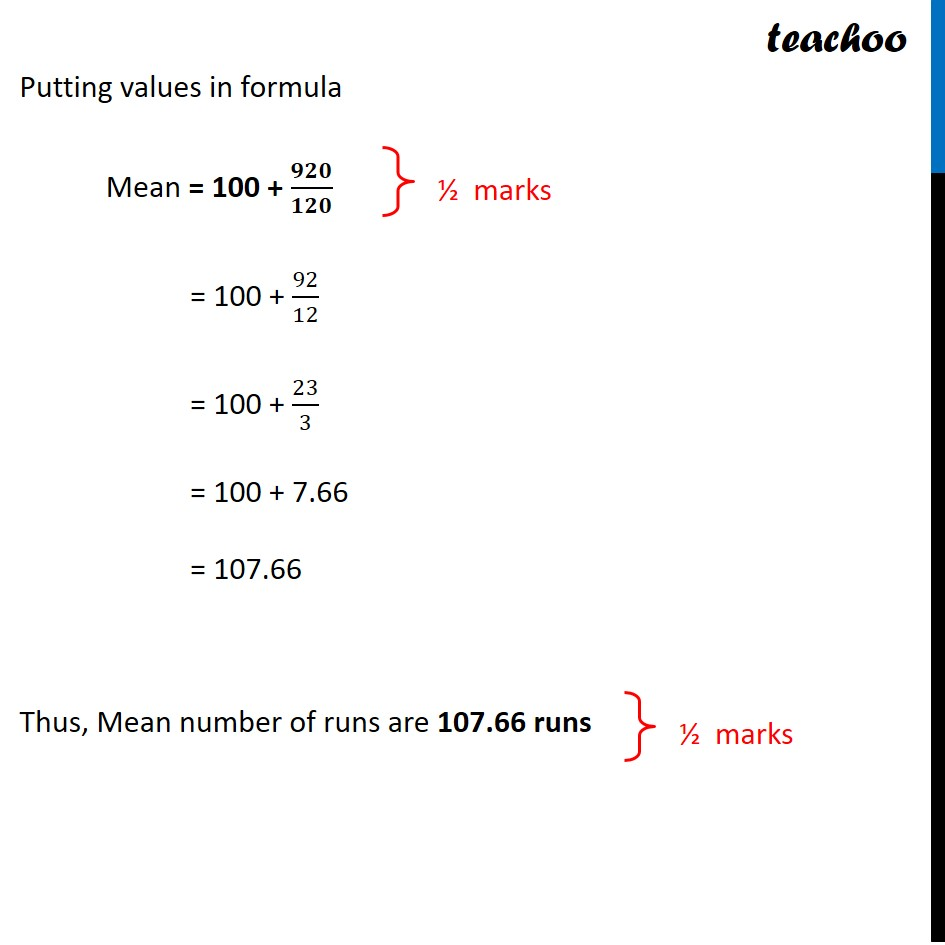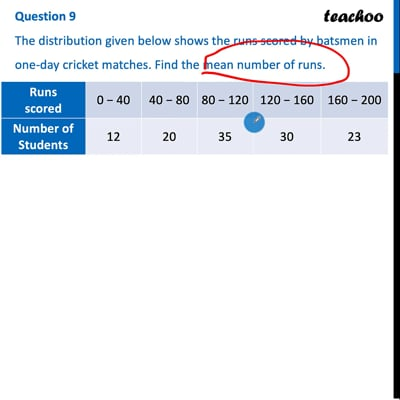CBSE Class 10 Sample Paper for 2022 Boards - Maths Standard [Term 2]

Class 10
Solutions of Sample Papers for Class 10 Boards

## The distribution given below shows the runs scored by batsmen in one-day cricket matches. Find the mean number of runs.

This question is similar to Ex 14.1, 3 Chapter 14 Class 10 - StatisticsThis video is only available for Teachoo black users

### Transcript

The distribution given below shows the runs scored by batsmen in one-day cricket matches. Find the mean number of runs. 20 – 100 = –80 23 × 80 = 1840 Mean(𝑥 ̅) = a + (∑▒𝒇𝒊𝒅𝒊)/(∑▒𝒇𝒊) Where a = Assumed Mean h = Class interval ½ marks = 40 − 0 = 40 Putting values in formula Mean = 100 + 𝟗𝟐𝟎/𝟏𝟐𝟎 = 100 + 92/12 = 100 + 23/3 = 100 + 7.66 = 107.66 Thus, Mean number of runs are 107.66 runs ½ marks ½ marks